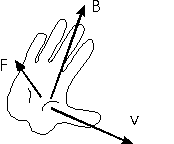The right-hand rule

The magnitude of the force felt by a charge q moving with velocity v through a magnetic field B is:As stated previously, the direction of the force is perpendicular to both v and B. One more thing must be said to define the direction. For example, if both v and B point in the plane of the screen, F could point either into or out of the screen to be perpendicular to both vectors. The flat right-hand rule is used to determine which of these two directions for F is the correct one.To find the direction of F,

1. Use your RIGHT hand (dropping pencil if necessary).
2. Extend the fingers and extend the thumb at right angles to them.
3. Make your thumb point in the direction of I or q v.
4. Make your fingers point in the direction of B.
5. The direction of the force is now given by the direction your palm would push something.
This version of the right hand rule applies directly to finding the direction of the force on a segment of wire carrying a current I. It also works to find the direction of the force on a moving charge; you only have to realize that for a positive charge I points in the direction of v, while for a negative charge, v is opposite to I.

Examples       Magnetic fields and forces index        Lecture index